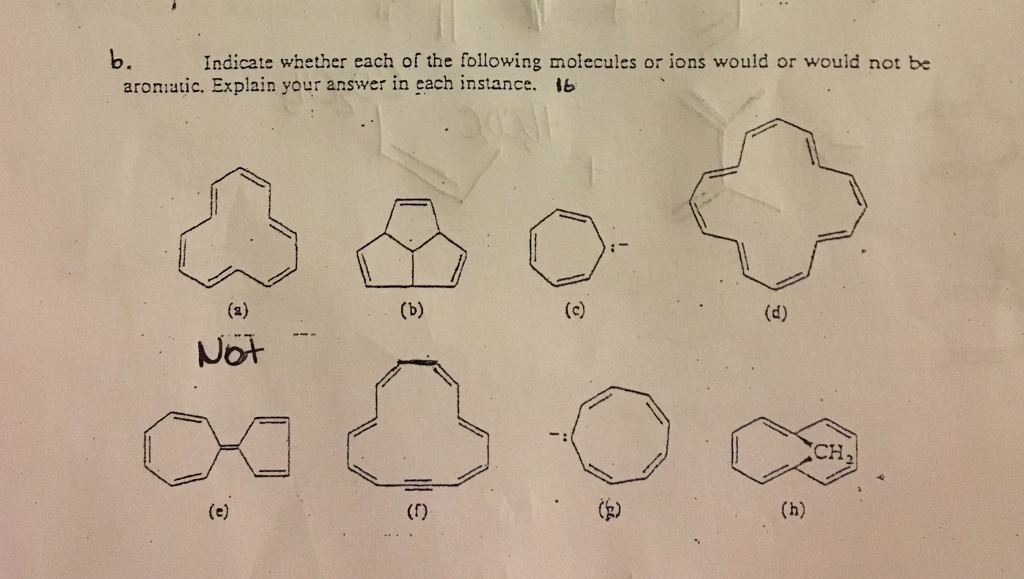# Question & Answer: Indicate whether each of the following molecules or ions would or would not be aromatic…..Indicate whether each of the following molecules or ions would or would not be aromatic. Explain your answer in each instance.

Rules for Aromaticity, Antiaromaticity and Nonaromaticity

A molecule is aromatic if:

Don't use plagiarized sources. Get Your Custom Essay on
Question & Answer: Indicate whether each of the following molecules or ions would or would not be aromatic…..
GET AN ESSAY WRITTEN FOR YOU FROM AS LOW AS \$13/PAGE

– It must be a PLANAR moelcule, i.e. 2-D; should not have a single sp3 hybridized carbon

– Must follow Huckel’s Rule, which state that the conjugated electrons must fit 4n+2 rule… that is

n (0,1,2,3,4…) then, total electrons E = (2, 6, 10, 14, 18…)

– Must be CYCLIC, so the electrons can actually flow in the “ring”

– Most of the species must be Sp2 hybridized (not sp3)

An antiaromatic species is similar to aromatic… it should only fail in:

– It must be a PLANAR moelcule, i.e. 2-D; should not have a single sp3 hybridized carbon

– Must NOT follow Huckel’s Rule, which state that the conjugated electrons must fit 4n+2 rule… that is

n (0,1,2,3,4…) then, total electrons E = (2, 6, 10, 14, 18…); if this is anti aromatic, the lone pairs should be all but those set of numbers…

– Must be CYCLIC, so the electrons can actually flow in the “ring”

– Most of the species must be Sp2 hybridized (not sp3)

Finally… NONAromatic are those that will fail at least other than huckels rule:

i.e.

If this molecule is NOT 2-D, i.e. not planar, then this is antiaromatic

If this is not a cycle, then this is NOT aromatic

There is any SP or SP3 hybridized…

Knowing this:

a)

this is cyclic, and 2-D

total electrons in conjugated system = 6 double bonds = 6×2 = 12 electrons

this does not fit hucke’s rule, therefore

antiaromatic

b)

This appears to be 2-Dimensional

but it is not, the central carbon is SP3 hybridized, therefore this is NONAROMATIC

c)

This must be planar, and SP2 hybridized

total electrons in conjguated system = 3xdouble bonds + lone pair = 3*2 + 1*2 = 6+2 = 8

this does NOT fit huckels rule, therefore, ANTIAROMATIC

d)

Planar, 2-D and sp2 hybridization

then

total double dbond s= 8; total electrons = 8*2 = 16

once again this fails huckel rule, so this must be antiaromatic

e)

this is planar, due to the double bonds between both rings

lone pairs = none

doulbe bonds = 6; therefore conjguated system is 6×2 =12

this is antiaroamtic

f)

double bonds = 6×2 = 12

triple bonds ( accounts for only 2 for cnojugated system) = 2

total electrons in system = 12+2 = 4

this applies and staisfies huckel rule

this is planar

therefore AROMATIC

g)

ttal lone pairs = 1

doulbe bonds = 4

total e- = 1×2 + 4×2 = 10

this is then AROMATIC

h)

this cant be aromatic, due to the CH2 present, i.e. SP3 hybridization

this is NONAROMATIC